Courses

# WBJEE Maths Test - 8

## 75 Questions MCQ Test WBJEE Sample Papers, Section Wise & Full Mock Tests | WBJEE Maths Test - 8

Description
This mock test of WBJEE Maths Test - 8 for JEE helps you for every JEE entrance exam. This contains 75 Multiple Choice Questions for JEE WBJEE Maths Test - 8 (mcq) to study with solutions a complete question bank. The solved questions answers in this WBJEE Maths Test - 8 quiz give you a good mix of easy questions and tough questions. JEE students definitely take this WBJEE Maths Test - 8 exercise for a better result in the exam. You can find other WBJEE Maths Test - 8 extra questions, long questions & short questions for JEE on EduRev as well by searching above.
QUESTION: 1

### In the expansion of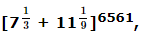the number of terms free from radicals is

Solution:

General term,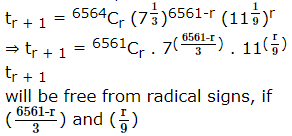are integers for
0 ≤ r ≤ 6561
∴ r = 0, 9, 18, 27, ...6561
0, 9, 18, ...6561 is in A.P.
First term, a = 0
Common - difference = 18 - 9 = 9
Last term, t = 6561
l = a + (n - 1)d, where n = number of terms
⇒ 6561 = 0 + (n - 1)9
⇒ 9(n - 1) = 6561
⇒ n - 1 = 729
⇒ n = 729 + 1
⇒ n = 730

QUESTION: 2

Solution:
QUESTION: 3

### The coefficient of x3 in ((√x5) + (3/√x3))6 is

Solution:
QUESTION: 4
Equation x2+2ax-b2=0 has real roots $\alpha$,$\beta$ and equation x2+2px-q2=0 has real roots $\gamma$,$\delta$. If the circle C is drawn with the points ($\alpha$,$\gamma$), ($\beta$,$\delta$) as extremities of a diameter, then the equation of C is
Solution:
QUESTION: 5

If the cube roots of unity are 1,ω,ω2 then the roots of the equation (x - 2)3+27 = 0 are

Solution:
QUESTION: 6

The equation of circle which passes through (4,5) and whose centre is (2,2) is

Solution:
QUESTION: 7
Let z1 and z2 be two complex numbers whose principle argument are α and β then, arg (z1.z2) =
Solution: Let z1 and z2 have polar representations z1 = r1( cos α + isinα)
and z2 = r2 (cosβ + isinβ ).
Then z1 z2 = r1 (cosα+i sinα) r2 (cosβ + isinβ )
= r1 r2 (cosαcosβ − sinαsinβ) + i(cosαsinβ + sinαcosβ )
= r1 r2 (cos (α+β ) + isin (α+β )),
which is the polar representation of z1 z2 , as r1 r2 = |z1 ||z2 |=|z1 z2 |.
Hence α+β is an argument of z1 z2
QUESTION: 8

If m and n are integers, then what is the value of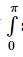sin mx sin nxdx . If m ≠ n

Solution:

Since sin mx, sin nx is an odd function if m ≠ n, then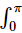sin mx . sin nx dx = 0

QUESTION: 9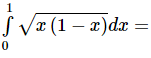Solution:
QUESTION: 10Solution:
QUESTION: 11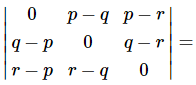Solution:
QUESTION: 12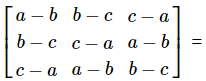Solution:
QUESTION: 13

The area bounded by the parabola y2=4ax and the straight line y=2ax is

Solution: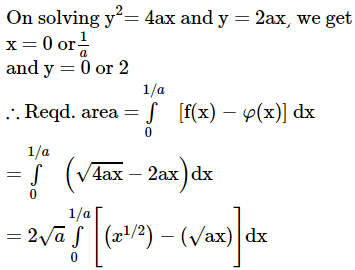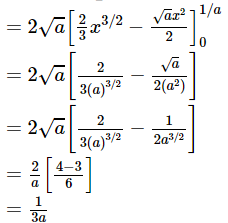QUESTION: 14

The solution of the differential equation 2xy(dy/dx)=x2+3y2 is (where c is a constant)

Solution:
QUESTION: 15

What is the solution of x2y2dy = (1−xy3) dx?

Solution:
QUESTION: 16

If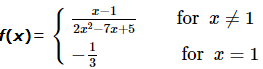then f ′(1) =

Solution:
QUESTION: 17

The curve represented by x = 2(cos t + sin t), y = 5 (cos t - sin t) is

Solution:
QUESTION: 18

The eccentric angles of the extremities of the latus-rectum intersecting positive x-axis of the ellipse ((x2/a2) + (y2/b2) = 1) are given by

Solution:
QUESTION: 19

If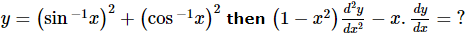Solution: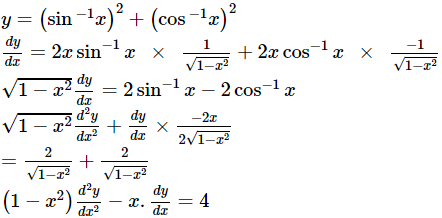QUESTION: 20

The slopes of the common tangents to the hyperbola x2/y - y2/16 = 1 and y2/9 - x2/16 = 1 are

Solution:
QUESTION: 21
The foci of the hyperbola 9x2 - 16y2 + 18x + 32y - 151 = 0 are
Solution:
QUESTION: 22

The function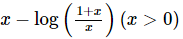is increasing in

Solution:
QUESTION: 23

The value of cos⁻1(cos 5π/3) + sin⁻1 (sin 5π/3) is

Solution:
QUESTION: 24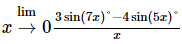is equal to

Solution: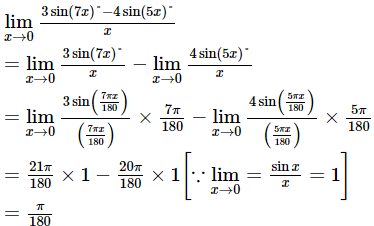QUESTION: 25

For all real x, the minimum value of (1 - x + x2)/(1 + x + x2) is

Solution:
QUESTION: 26

The system of linear equation x + y + z = 2, 2x + y - z = 3, 3x + 2y + kz = 4 has a unique solution, then

Solution:

Augmented matrix is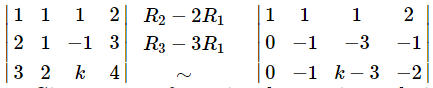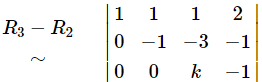∴ Given system of equations has a unique solution ⇒ k ≠ 0

QUESTION: 27
If the vertices O,A,B of any equilateral triangle are situated at z=0, z=z₁ and z=z₂ respectively, then which of the following are true ?
Solution:
QUESTION: 28
Which of the following points lie on the parabola x2=4ay ?
Solution:
QUESTION: 29
P,Q,R and S have to deliver lecture, then in how many ways can the lectures be arranged?
Solution: Total no. of ways delivering lectures = 4P4 = 4! = 24
QUESTION: 30

The length of the normal chord to the parabola y2 = 4x which subtends a right angle at the vertex is

Solution: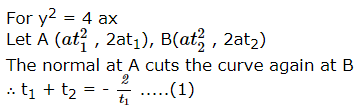Again AB subtends a right angle at the vertex O (0, 0) of the parabola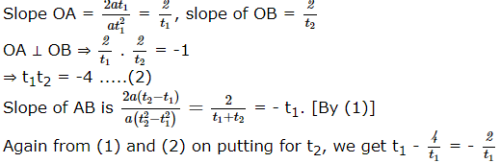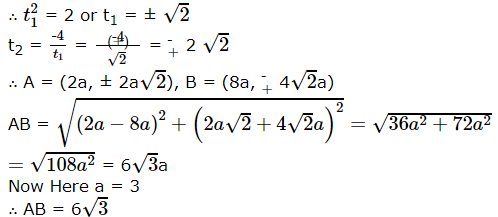QUESTION: 31
In how many different ways can the letters of the word 'AUCTION' be arranged so that the vowels always come together?
Solution:
QUESTION: 32

If 20Cn+2 = nC16, then n =

Solution: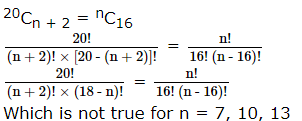QUESTION: 33
A determinant is chosen at random from the set of all determinants of order 2 with elements 0 or 1 only. The probability that the determinant chosen is non-zero is
Solution:
QUESTION: 34

What is the chance that a leap year should have 53 sundays?

Solution:
QUESTION: 35

A problem in EAMCET examination is given to 3 students A, B and C whose chances of solving it are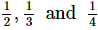respectively. The probability that the problem will be solved is

Solution:
QUESTION: 36

f(x) = 1 + 2 sin x + 3 cos2x, 0 ≤ x ≤ (2π/3) is

Solution:
QUESTION: 37

In ΔABC ,(a + b + c)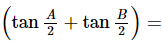Solution: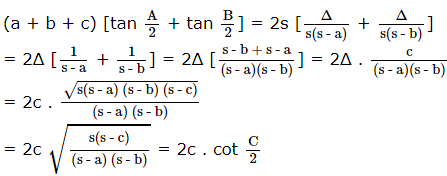QUESTION: 38

If R denotes circumradius then in a ΔABC ,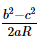is equal to

Solution: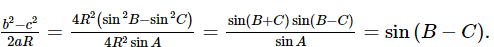QUESTION: 39

If the roots of x2 + x + a = 0 exceed a, then

Solution:
QUESTION: 40

If f(x) is continuous and differentiable over [−2, 5] and −4 ≤ f′ (x) ≤ 3 for all x in (−2, 5) then the greatest possible value of f(5) − f(−2), is

Solution: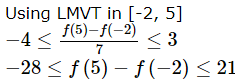QUESTION: 41

If y = x - x2 + x3 - x4 + ... to ∞, then the value of x will be (-1 < x < 1)

Solution:
QUESTION: 42

The sum of an infinite number of G.P. is 20, and the sum of their squares is 100. The first term of the G.P. is

Solution: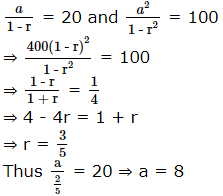QUESTION: 43
The two geometric means between 1 and 64 are
Solution:
QUESTION: 44
If a, b, c of a triangle are in A.P., then cot C/2 =
Solution:
QUESTION: 45
Let A = {a,b,c}, B = {b,c,d}, C = {a,b,d,e}, then A ∩ (B ∪ C) is
Solution:
QUESTION: 46

The equation of bisectors between the lines 3x+4y-7=0 and 12x+5y+17=0 are

Solution:
QUESTION: 47
If f(x) = (x - 3)/(x + 1), then f [f{f(x)}] =
Solution:
QUESTION: 48

The equations of two lines which pass through the point (3,2) and make angle of 45º with the line x-2y=3 are

Solution:
QUESTION: 49

The roots of the equation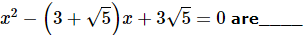Solution:

Given equations is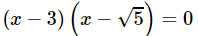QUESTION: 50

Solution of 7 sin2x + 3 cos2 x = 4 is

Solution:
QUESTION: 51

If α1 , α2 , α3 , … … , α n are the nth roots of unity, then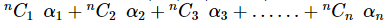equals

Solution:
QUESTION: 52

If the hypotenuse of right angled triangle is four times the length of perpendicular drawn from opposite vertex to it, then the difference of two acute angle will be

Solution:
QUESTION: 53

ABC is an isosceles triangle with AB = AC. If B (1, 3), C(- 2, 7) then vertex A may be

Solution:
QUESTION: 54

The area bounded by the curve f(x) = x + sinx and its inverse function between the ordinates x = 0 to x = 2 π is

Solution:
QUESTION: 55

Area bounded by the curve y = sin-1 |(sinx)| and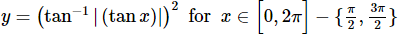Solution:
QUESTION: 56

Let f (x) = ex sin x, be the equation of a given curve. If at x = a, 0 ≤ a ≤ 2 π , the slope of the tangent is the maximum, then the value of a is

Solution:
QUESTION: 57

For real x, the function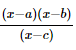will assume all real values provided :

Solution:
QUESTION: 58

The ellipse x2+4y2=4 is inscribed in a rectangle aligned with the coordinate axes, which in turn is inscribed in another ellipse that passes through the point(4, 0). Then the equation of the ellipse is:

Solution:
QUESTION: 59

n non-zero real numbers (n ≥ 2) are written on a board. Ritu erases any two numbers, say a and b, and then writes the numbers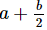and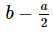instead. Then which of the following is true?

Solution:
QUESTION: 60

If a ∗ b = a+b-2 and if x ∗ 3 = 7 then what is the value of x-1?

Solution:
QUESTION: 61

If a polynomial g(x) satisfies x g(x + 1) = (x - 3)g(x) for all x and g(3) = 6, then the value of g(25) is

Solution:
QUESTION: 62

If ∫ tan7 xdx = f (x) + log | cos x | then

Solution:
QUESTION: 63

Three roots of the equation, x4 − px3 + qx2 − rx + s = 0 are tan A, tan B and tan C where A, B, C are the angles of a triangle. The fourth root of the biquadratic is:

Solution:
QUESTION: 64

If Ik means logloglog....logx, the log being repeated K times, then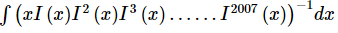is equal to

Solution:
QUESTION: 65

The value of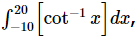, where [.] is greatest integer function is

Solution:
*Multiple options can be correct
QUESTION: 66

Which of the following function(s) from f : A → A are not invertible, where A=[-1,1]:

Solution:
*Multiple options can be correct
QUESTION: 67

A tangent is drawn at point P (x1 , y1) on the hyperbola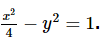If pair of tangents are drawn from any point on this tangent to the circle x2 + y2 = 16 such that chords of contact are concurrent at the point ( x2 , y2 ) then

Solution:
*Multiple options can be correct
QUESTION: 68

A particle is moving in a straight line such that its distance at any time t is given by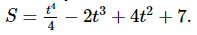Then

Solution:
*Multiple options can be correct
QUESTION: 69

Let f (x)   = sin x + cos x be defined in [0 , 2π] , then f (x)

Solution:
*Multiple options can be correct
QUESTION: 70

If the tangents drawn from the point (0, 2) to the parabola y2 = 4ax are inclined at angle 3 π 4 , then the value of 'a' is

Solution:
*Multiple options can be correct
QUESTION: 71

Circles are drawn on chords of the rectangular hyperbola xy = c2 parallel to the line y = x as diameters. All such circles pass through two fixed points whose co-ordinates are

Solution:
*Multiple options can be correct
QUESTION: 72

In the triangle ABC, the altitude, angle bisector and median from C divide the angle C into four equal angles. Then which of the following statements is true?

Solution:
*Multiple options can be correct
QUESTION: 73

If the line ax + by + c = 0 is a normal to the hyperbola xy = 1, then

Solution:
*Multiple options can be correct
QUESTION: 74

The value of x satisfying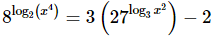are

Solution:
*Multiple options can be correct
QUESTION: 75

If M ans N are two events, the pobability that exactly one of them occurs is

Solution: